# DATA MODELChapter I - A Survey of Bayesian Data Mining Data Mining: Opportunities and Challenges by John Wang (ed) Idea Group Publishing 2003Brought to you by Team-Fly

## DATA MODEL

We consider a data matrix where rows are cases and columns are variables. In a medical research application, the row could be associated with a person or an investigation (patient and date). In an Internet use application, the case could be an interaction session. The columns describe a large number of variables that could be recorded, such as background data (occupation, gender, age, etc.), and numbers extracted from investigations made, like sizes of brain regions, receptor densities and blood flow by region, etc. Categorical data can be equipped with a confidence (probability that the recorded datum is correct), and numerical data with an error bar. Every datum can be recorded as missing, and the reason for missing data can be related to patient condition or external factors (like equipment unavailability or time and cost constraints). Only the latter type of missing data is (at least approximately) unrelated to the domain of investigation. On the level of exploratory analysis, we confine ourselves to discrete distributions, with Dirichlet priors. If the data do not satisfy the conditions following this approach, (e.g., non-discreteness for real valued variable), they may do so after suitable transformation, discretization, and/or segmentation.

In many applications the definition of the data matrices is not obvious. For example, in text-mining applications, the character sequences of information items are not directly of interest, but a complex coding of their meaning must be done, taking into account the (natural) language used and the purpose of the application.

### Multivariate Data Models

Given a data matrix, the first question that arises concerns the relationships between its variables (columns). Could some pairs of variables be considered independent, or do the data indicate that there is a connection between them either directly causal, mediated through another variable, or introduced through sampling bias? These questions are analyzed using graphical models, directed or decomposable (Madigan & Raftery, 1994). As an example, in Figure 1, M1 indicates a model where A and B are dependent, whereas they are independent in model M2. In Figure 2, we describe a directed graphical model M4′′ indicating that variables A and B are independently determined, but the value of C will be dependent on the values for A and B. The similar decomposable model M4 indicates that the dependence of A and B is completely explained by the mediation of variable C.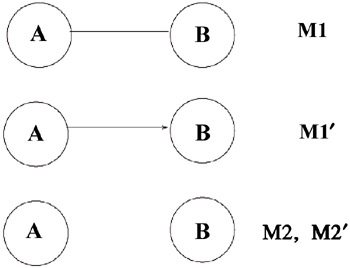Figure 1: Graphical models, dependence or independence?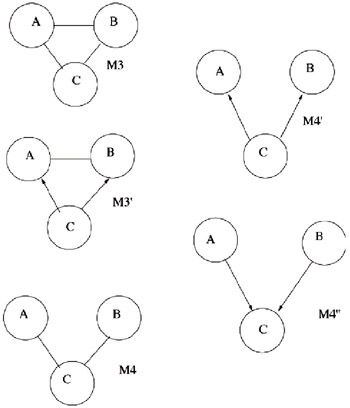Figure 2: Graphical models, conditional independence?

Bayesian analysis of graphical models involves selecting all or some graphs on the variables, dependent on prior information, and comparing their posterior probabilities with respect to the data matrix. A set of highest posterior probability models usually gives many clues to the data dependencies, although one must as always in statistics constantly remember that dependencies are not necessarily causalities.

A second question that arises concerns the relationships between rows (cases) in the data matrix. Are the cases built up from distinguishable classes, so that each class has its data generated from a simpler graphical model than that of the whole data set? In the simplest case, these classes can be directly read off in the graphical model. In a data matrix where intervariable dependencies are well explained by the model M4, if C is a categorical variable taking only few values, splitting the rows by the value of C could give a set of data matrices in each of which A and B are independent. However, the interesting cases are those in which the classes cannot be directly seen in a graphical model. If the data matrix of the example contained only variables A and B, because C was unavailable or unknown to interfere with A and B, the highest posterior probability graphical model is one with a link from A to B. The classes would still be there, but since C would be latent or hidden, the classes would have to be derived from only the A and B variables. A different case of classification is one in which the values of one numerical variable are drawn from several normal distributions with different means and variances. The full column would fit very badly to any single normal distribution, but after classification, each class could have a set of values fitting well to a class-specific normal distribution. The problem of identifying classes is known as unsupervised classification. A comprehensive system for classification based on Bayesian methodology is described in Cheeseman and Stutz (1995). A third question often the one of highest practical concern is whether some designated variable can be reliably predicted in the sense that it is well related to combinations of values of other variables, not only in the data matrix, but also with high confidence in new cases that are presented. Consider a data matrix well described by model M4 in Figure 2. It is conceivable that the value of C is a good predictor of variable B, and better than A. It also seems likely that knowing both A and C is of little help compared to knowing only C, because the influence of A on B is completely mediated by C. On the other hand, if we want to predict C, it is very conceivable that knowing both A and B is better than knowing only one of them.

### Bayesian Analysis and Over-Fitting

A natural procedure for estimating dependencies among categorical variables is by means of conditional probabilities estimated as frequencies in the data matrix. Such procedures usually lead to selection of more detailed models and give poor generalizing performance, in the sense that new sets of data are likely to have completely different dependencies. Various penalty terms have been tried to avoid over-fitting. However, the Bayesian method has a built-in mechanism that favors the simplest models compatible with the data, and also selects more detailed models as the amount of data increases. This effect appears, e.g., in the coin tossing example, where few tosses cannot give a verdict that the coin is unfair. The procedure is to compare posterior model probabilities, where the posterior probability of a model is obtained by combining its prior distribution of parameters with the probability of the data as a function of the parameters, using Bayes' rule. Thus, if p(Θ) is the prior pdf of the parameter (set) Θ of model M, and the probability of obtaining the case (row of data matrix) d is p(dM, Θ), then the probability in model M of the data matrix D containing the ordered cases di is: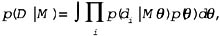and the posterior probability of model M given the data D is, by Bayes' rule: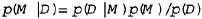Two models M1 and M2 can now be related with respect to the data by the Bayes factor p(D M1)/p(DM2) used to go from the prior to the posterior odds. With the Bayesian method, there is no need to penalize more detailed models to avoid over-fitting if M2 is more detailed than M1 in the sense of having more parameters to fit, then the parameter dimension is larger in M2, and p(Θ1) is larger than p(Θ2), which automatically penalizes M2 against M1 when the parameters are integrated out. This automatic penalization has been found appropriate in most application cases, and should be complemented by explicit prior model probabilities only when there is concrete prior information that justifies it or when the data is too abundant to select a model simple enough to comprehend. When the more detailed model is the true one, Bayes factor in favor of it will increase exponentially with sample size, but in the other case the Bayes factor in favor of the less detailed model will only increase polynomially.

 Brought to you by Team-Fly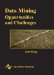Data Mining: Opportunities and Challenges
ISBN: 1591400511
EAN: 2147483647
Year: 2003
Pages: 194
Authors: John Wang